In the auto theming article, we learned that calling thematic_on() with no arguments applies auto coloring and that calling thematic_on(font = "auto") adds in automatic fonts. After reading that article, you may be left thinking, “What if auto theming doesn’t theme stuff exactly the way I want it to?” This article helps address that question by demonstrating how to do customized “high-level” theming with thematic as well as “lower-level” theming targeted specifically at ggplot2, lattice, and base graphics. In other words, we’ll first learn how to use thematic’s theming interface to set global defaults, then learn how to override those global defaults in plot-specific code.

## Theming with thematic

### Main colors

From the function signature of thematic_on(), we can see the three main colors of a thematic theme, which all default to a special value of "auto".

thematic_on(
bg = "auto", fg = "auto", accent = "auto", font = NA,
sequential = sequential_gradient(), qualitative = okabe_ito()
)

However, these arguments also accept any valid R (or CSS) color string. So, to “opt-out” of auto coloring, just specify the colors:

library(ggplot2)
thematic_on(bg = "#222222", fg = "white", accent = "purple")
ggsmooth <- ggplot(mtcars, aes(wt, mpg)) + geom_point() + geom_smooth()
ggsmooth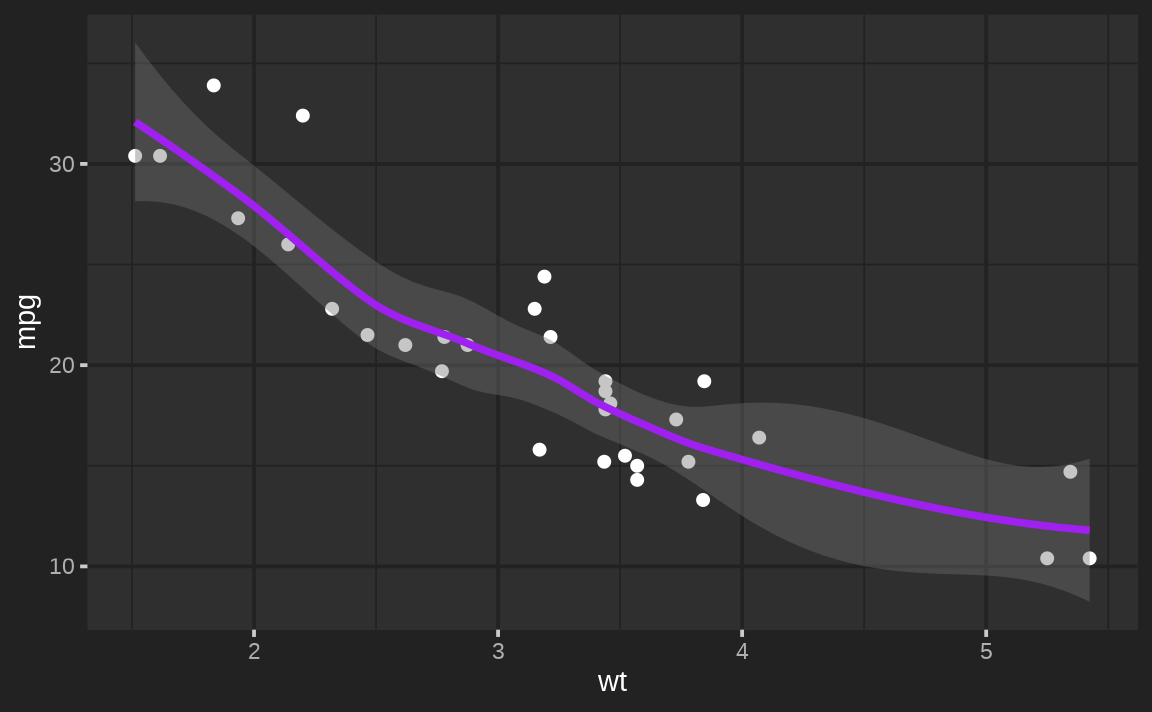Similar to how font = NA prevents thematic from changing any font defaults, accent = NA prevents accent color defaults (e.g., geom_smooth()’s color) from changing.

thematic_on(bg = "#222222", fg = "white", accent = NA)
ggsmooth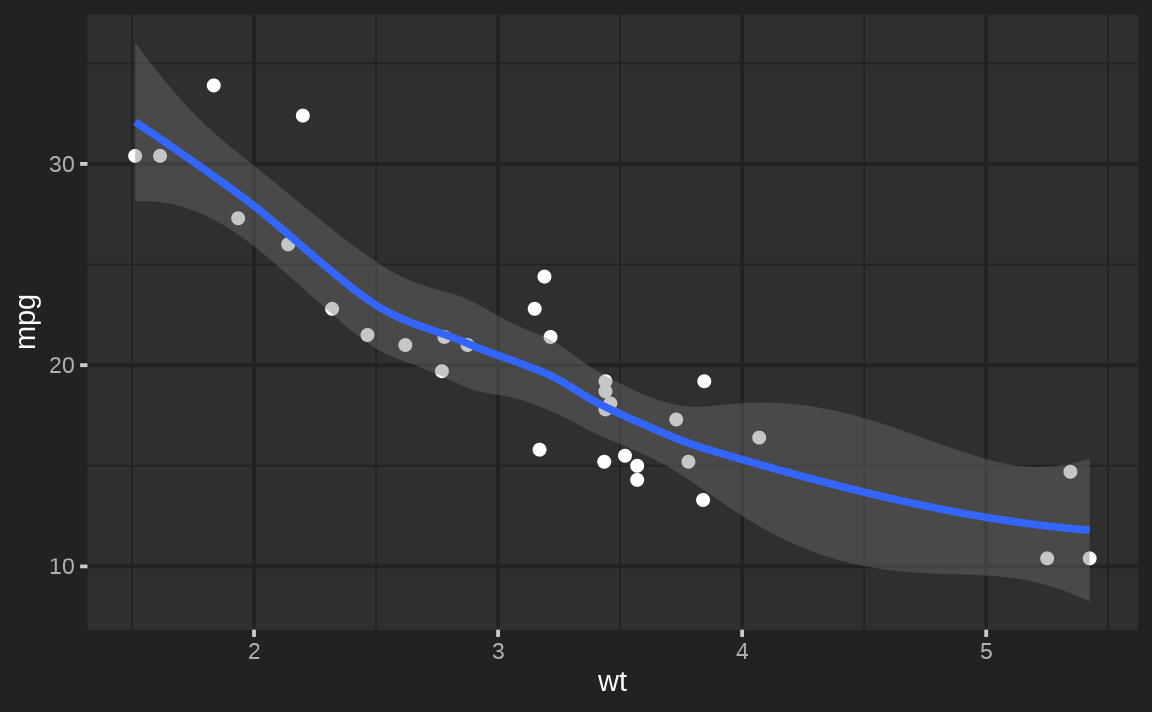### Sequential colorscales

The sequential argument is used to set a new default for sequential color scales (i.e., scale_fill_continuous() & scale_color_continuous()). Its default value, sequential_gradient(), defines a color gradient of fg -> accent -> bg:

thematic_on(bg = "#222222", fg = "white", accent = "purple")
ggcontour <- ggplot(faithfuld, aes(waiting, eruptions, z = density)) +
geom_raster(aes(fill = density)) +
geom_contour()
ggcontourTo flip the direction of the gradient to bg -> accent -> fg

thematic_on(
bg = "#222222", fg = "white", accent = "purple",
)
ggcontour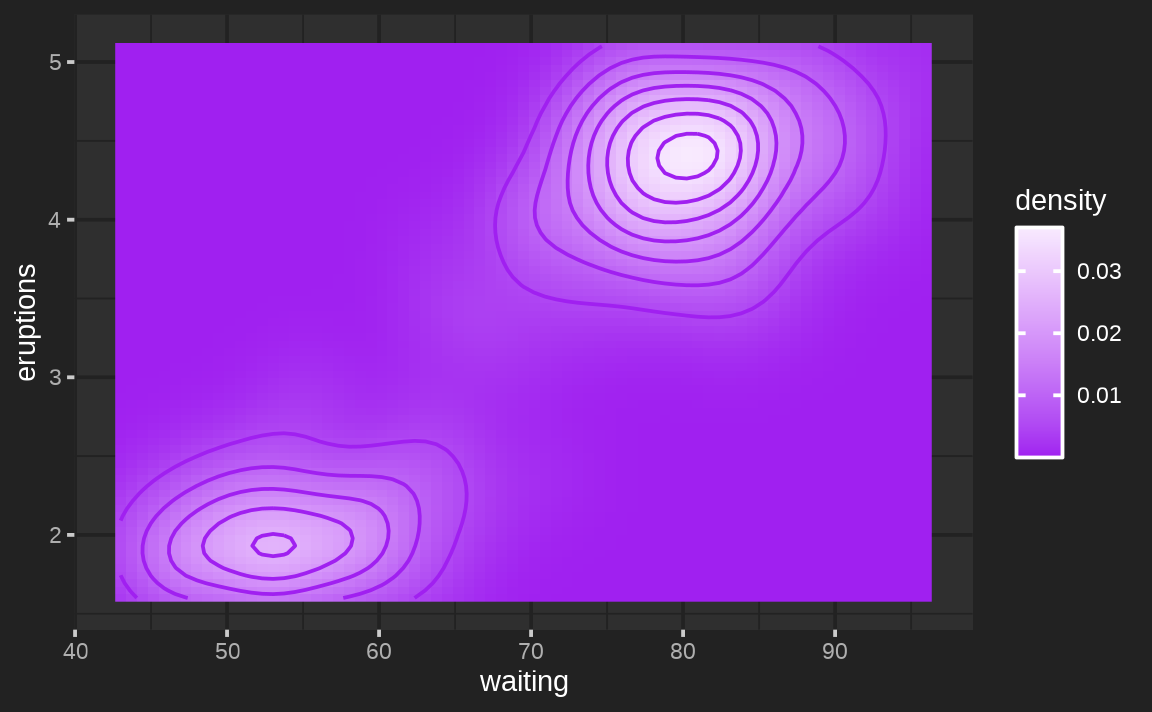If you look carefully, the endpoints of the gradient actually use a mixture of bg/fg and accent. The weighting of those mixtures can also be controlled via fg_weight and bg_weight. Here we make sure the gradient’s starts at the bg color and ends at the accent.

thematic_on(
bg = "#222222", fg = "white", accent = "purple",
sequential = sequential_gradient(fg_low = FALSE, fg_weight = 0, bg_weight = 1)
)
ggcontour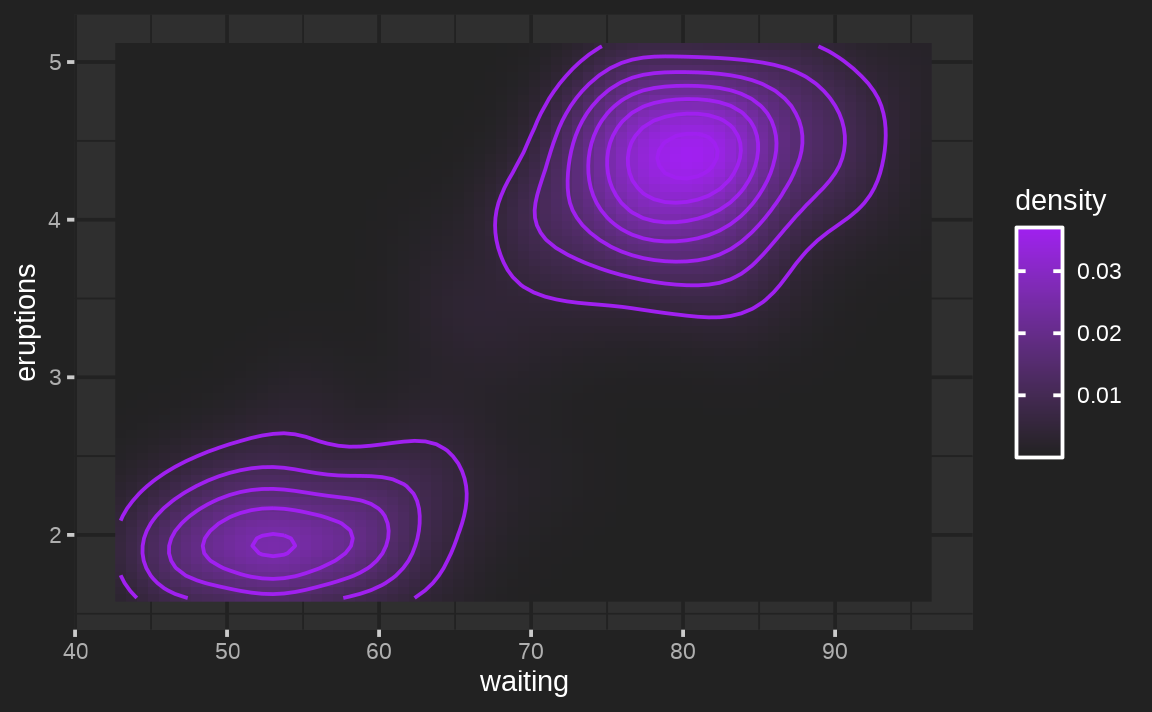If you don’t want sequential to be based on accent/bg/fg, you can also provide a vector of color codes defining the gradient.

thematic_on(bg = "#222222", fg = "white", accent = "white", sequential = hcl.colors(10))
ggcontourSimilar to how we opted out of new accent defaults, we can also opt-out of new sequential defaults

thematic_on(bg = "#222222", fg = "white", accent = "white", sequential = NA)
ggcontour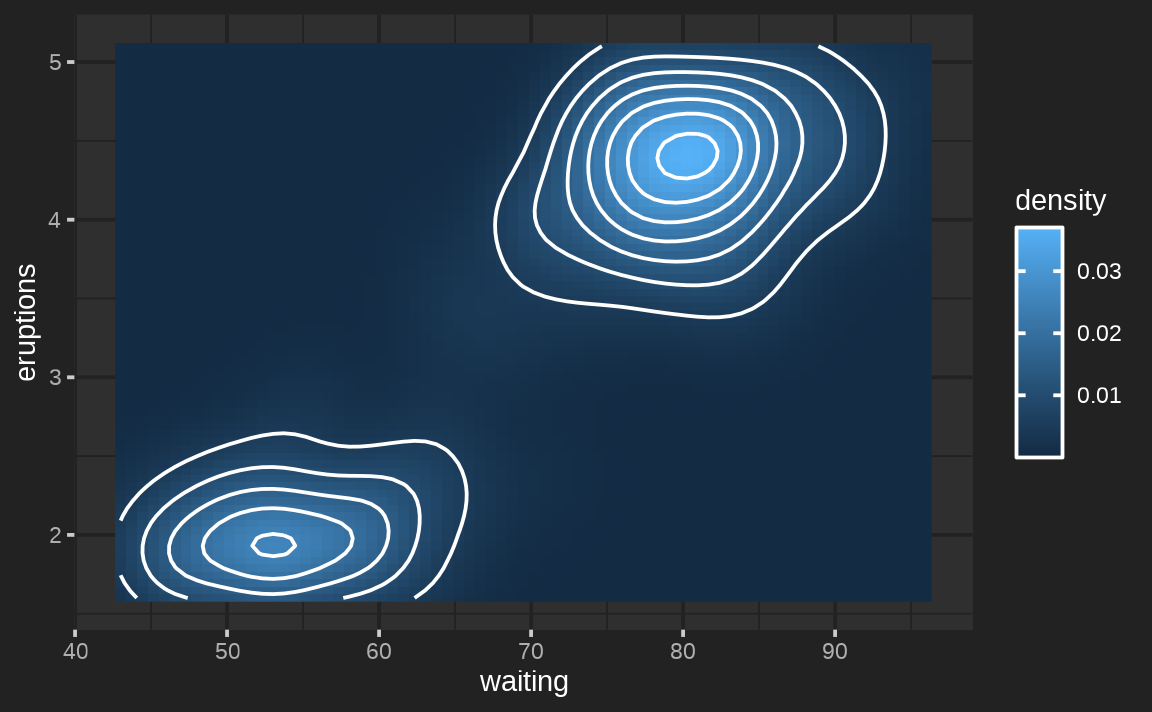### Qualitative colorscales

In addition to sequential colorscales, thematic also sets new defaults for qualitative (i.e., discrete) color scales based on the qualitative argument. The default, okabe_ito(), is a great color-blind safe option, but qualitative can be set to any vector of color codes. Here’s another good color-blind safe palette from https://colorbrewer2.org/#type=qualitative&scheme=Dark2

thematic_on(
bg = "#222222", fg = "white", qualitative = RColorBrewer::brewer.pal(8, "Dark2")
)
ggplot(mtcars, aes(wt, mpg, color = factor(cyl))) + geom_point()
#> Warning: thematic was unable to resolve accent='auto'. Try providing an
#> actual color (or NA) to the accent argument of thematic_on(). By the way,
#> 'auto' is only officially supported in shiny::renderPlot(), some rmarkdown
#> scenarios (specifically, html_document() with theme!=NULL), in RStudio, or
#> if auto_config_set() is used.In the event that a ggplot2 plot requires more colors than provided, it will fallback to the usual scale_[color/fill]_hue() behavior:

ggplot(mtcars, aes(wt, mpg, color = row.names(mtcars))) + geom_point()However, for base and lattice graphics, the qualitative colorscale (i.e., palette()) is recycled:

with(mtcars, plot(wt, mpg, col = seq_len(nrow(mtcars))))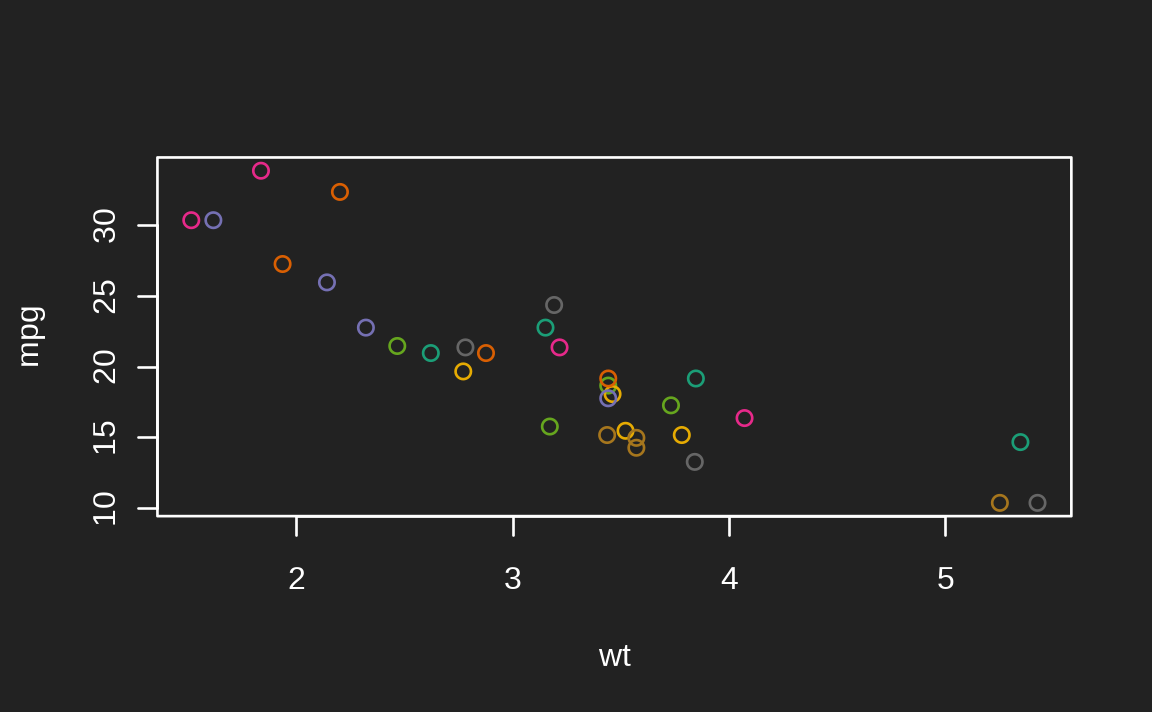Again, to prevent thematic from setting any new defaults for qualitative colorscales, provide an NA value

thematic_on(
bg = "#222222", fg = "white", qualitative = NA
)
ggplot(mtcars, aes(wt, mpg, color = factor(cyl))) + geom_point()### Fonts

The font argument accepts a character string specifying either a font already know to R or a Google Font. When a (new) Google Font is requested, thematic can automatically download, register, and cache (prior to plotting) so that Google Fonts “just work” if the showtext package is installed. In some cases, some additional setup may be required to get Google Fonts rendering properly — see the fonts article for more info.

thematic_on(bg = "#222222", fg = "white", font = "Oxanium")
ggsmoothfont also accepts a font_spec() object which, among other things, makes it easy to multiply all the font sizes by a scalar multiple:

thematic_on(bg = "#222222", fg = "white", font = font_spec("Oxanium", scale = 2))
ggsmooth## Theming with ggplot2

### Complete themes

Complete ggplot2 themes are theme() objects that fully specify every possible theme() element. The default ggplot2 theme, theme_gray(), is a complete theme, and ggplot2 provides some other useful complete themes such as theme_bw(), theme_minimal(), and theme_classic(). As we saw in the auto theming article, thematic can play nicely with complete themes so long as they’re set globally. This is because plot-specific code overrides the defaults that thematic sets, and so by adding a complete theme to a plot (i.e., ggsmooth + theme_classic()) it would override all the theme()-specific defaults set by thematic, which likely isn’t what you want.

ggplot2::theme_set(ggplot2::theme_classic())
thematic_on(bg = "#222222", fg = "white")
ggsmooth
# Now go back to the default
ggplot2::theme_set(ggplot2::theme_gray())Other ggplot2 extension packages such as ggthemes also provide useful complete themes. The image below links to a Shiny app that allows you to preview how various ggthemes themes look both before and after thematic in a Shiny app with some custom CSS.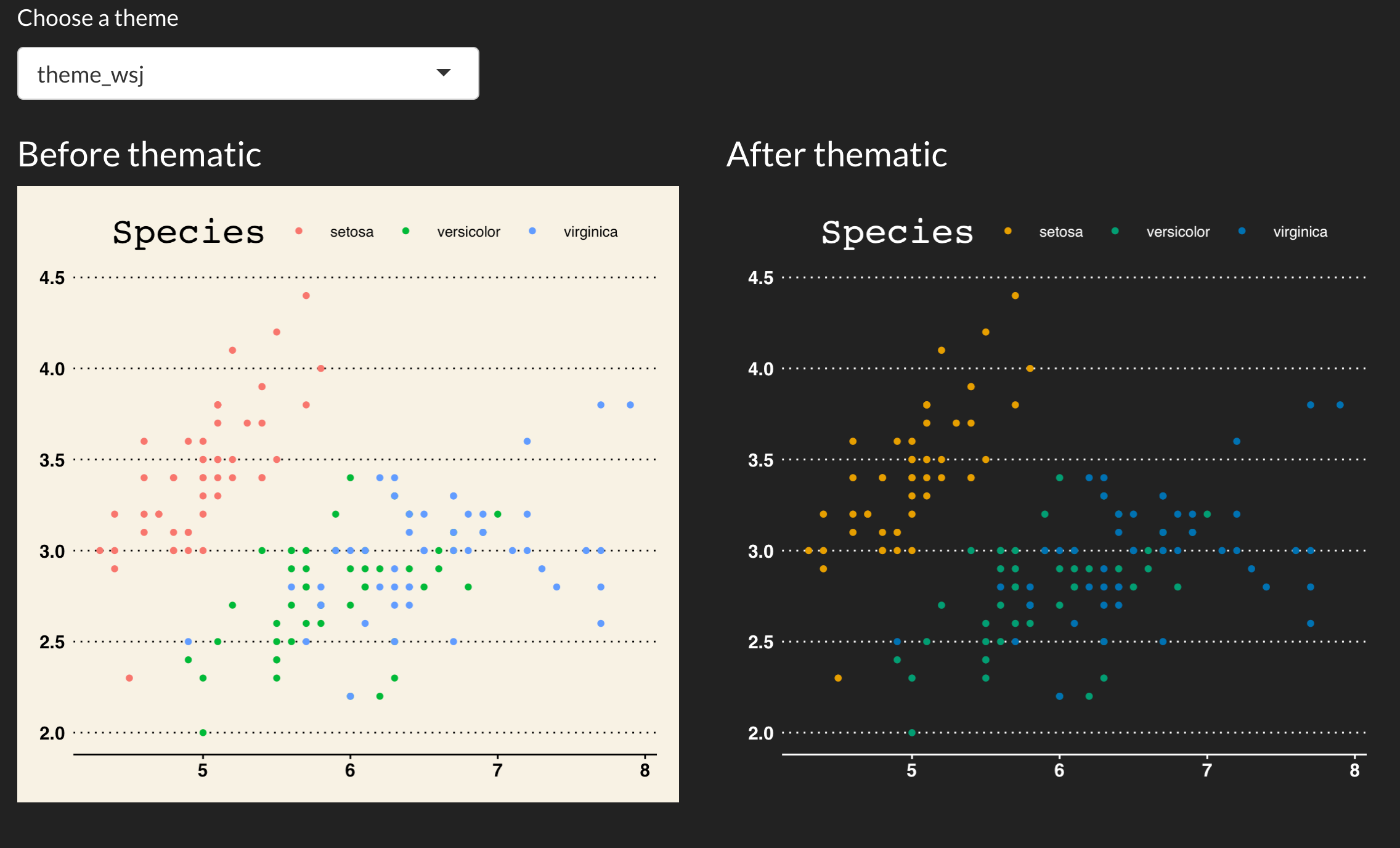### Partial themes

thematic_on() sets a new “complete” theme() default based on bg, fg, and font (based on a modified version of theme_gray()). Since this theme is “complete”, you probably don’t want to mix it with other complete themes (e.g., theme_bw()), but you can definitely override particular aspects with theme().

thematic_on(bg = "#222222", fg = "white")
p <- ggplot(mtcars, aes(wt, mpg)) +
geom_point() +
facet_wrap(~cyl)
p + theme(strip.background = element_rect(fill = "purple"))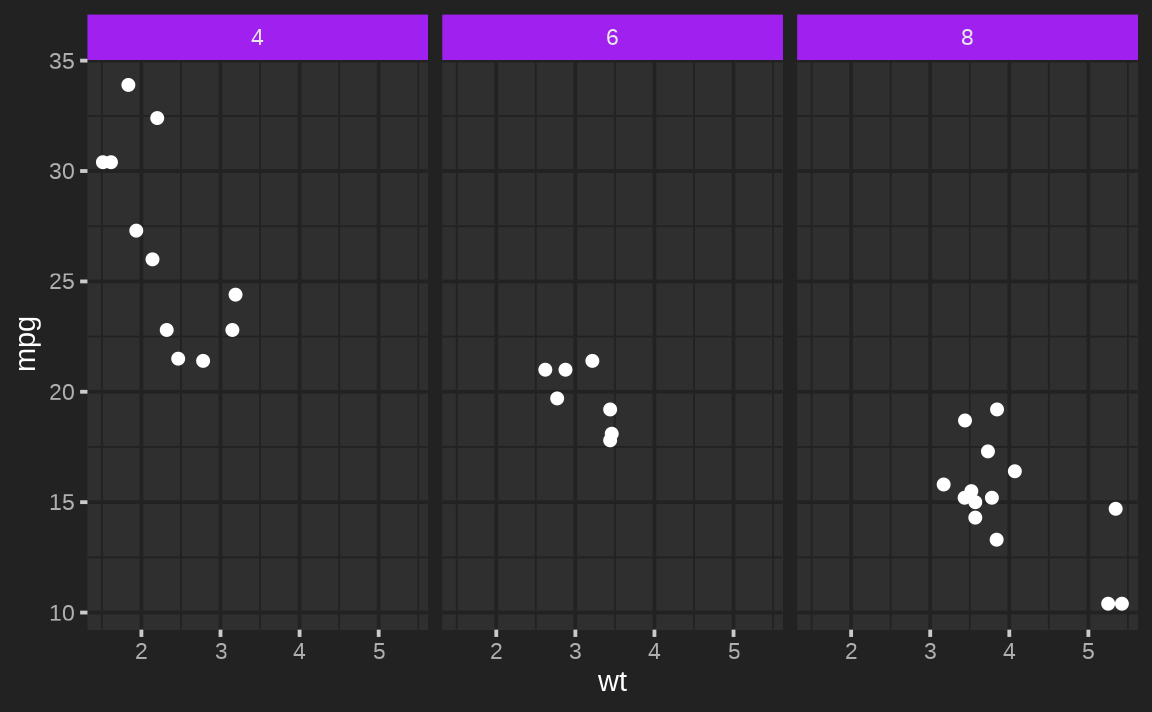By the way, it’s worth noting that thematic uses a fairly arbitrary amount of mixture between the fg and bg to set the theme. If you wanted different mixture(s) of fg and bg, then thematic_get_mixture() is useful:

my_theme <- theme(
panel.background = element_rect(fill = thematic_get_mixture(0.6)),
strip.background = element_rect(fill = thematic_get_mixture(0.3)),
strip.text = element_text(color = thematic_get_mixture(1))
)
p + my_theme### Geom defaults

For each relevant Geom (e.g., GeomPoint), new Geom$default_aes defaults are set (based on bg, fg, accent, and font). Grayscale colors (e.g., GeomPoint$default_aes$color) are assigned a mixture of bg and fg whereas non-grayscale colors (e.g., GeomSmooth$default_aes\$color) are assigned the accent color. It’s important to note these are just global defaults that only take effect if the aesthetic hasn’t been specified:

ggplot(mtcars, aes(wt, mpg)) +
geom_point(color = "red") +
facet_wrap(~cyl)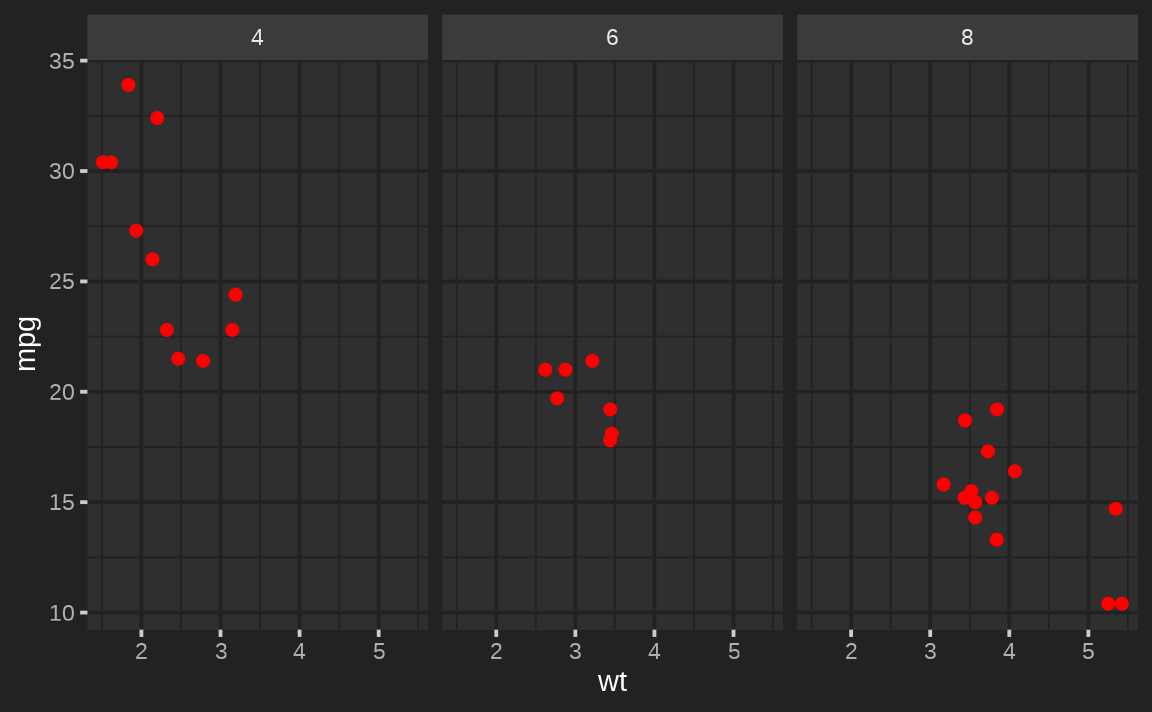### Scale defaults

thematic_on() sets a new scale_fill_continuous() and scale_color_continuous() defaults (based on sequential). See the previous section for an extensive discussion on how sequential works and note that adding a relevant (continuous) scale renders sequential irrelevant:

ggplot(faithfuld, aes(waiting, eruptions, z = density)) +
geom_raster(aes(fill = density)) +
scale_fill_gradient2(midpoint = 0.02)It also defines new scale_fill_discrete() and scale_color_discrete() defaults (based on qualitative). As with sequential colorscales, adding a relevant (discrete) scale renders qualitative irrelevant:

ggplot(economics_long) +
geom_line(aes(date, value01, color = variable)) +
scale_color_brewer(type = "qual")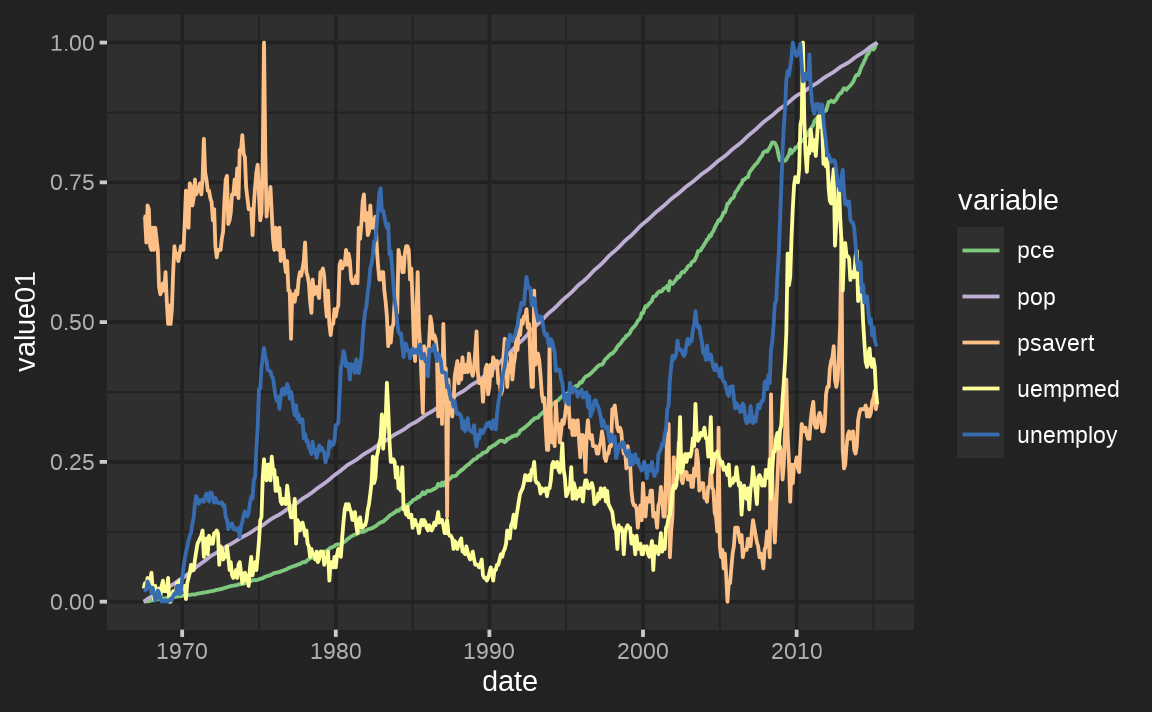### Third party extensions

As a side note, when it comes to third party ggplot2 extension packages, thematic should work as expected (let us know if it doesn’t) as long as those extension packages aren’t hard coding defaults in un-expected ways.

library(GGally)
ggpairs(iris, aes(color = Species))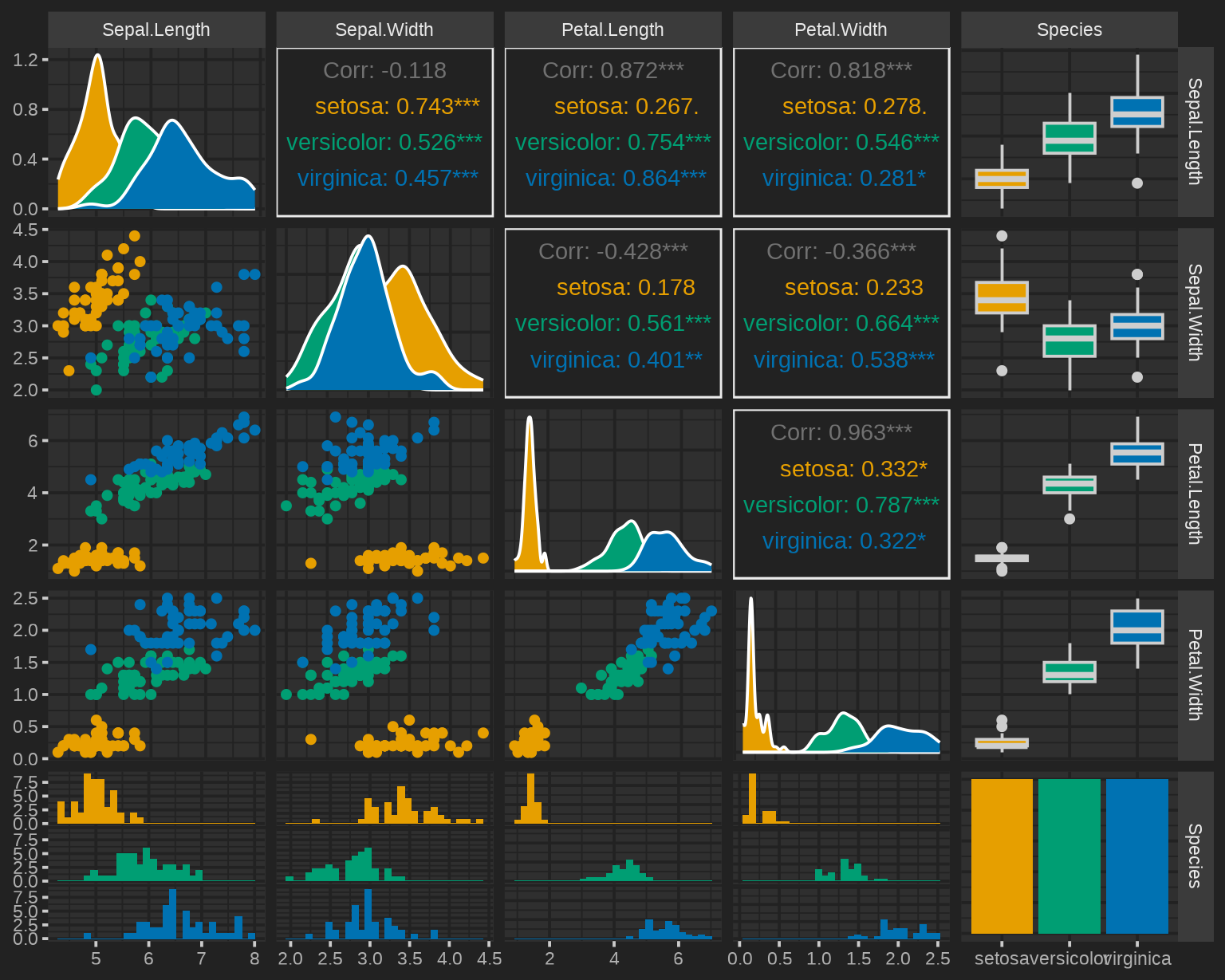## Theming with lattice

thematic also works with lattice; however, beware that theming decisions are made so that lattice plots look somewhat similar to ggplot2 (i.e. panel background is a mixture of bg and fg instead of just bg). Also, similar to base graphics, lattice doesn’t have a global distinction between a qualitative and sequential colorscales, so sequential isn’t used in lattice. Instead, for consistency with lattice’s default, the “regions” colorscale interpolates between qualitative, bg, and qualitative.

(Btw, for lattice, accent may be of length 2. The first is used for ‘stroke’ and the second for ‘fill’).

# accent may be of length 2 (stroke and fill)
thematic_on(bg = "#222222", fg = "white", accent = c("purple", "red"))
library(lattice)
show.settings()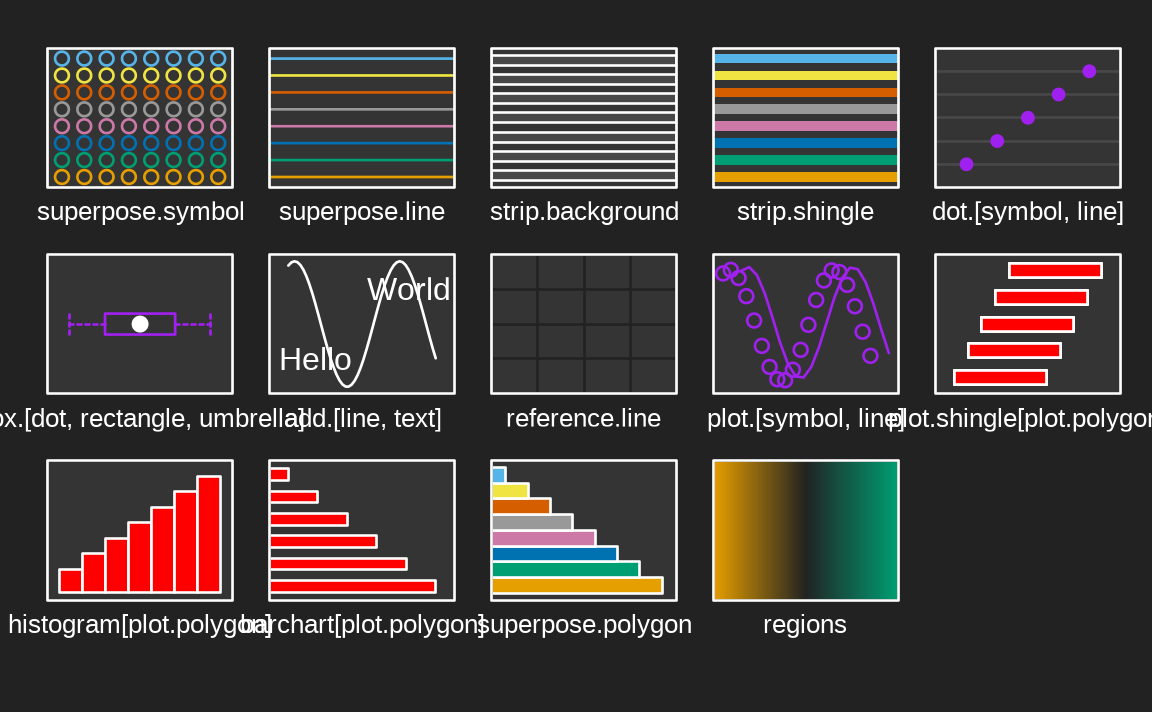It might seem strange to have bg define the middle of the color gradient, but it’s intentional so that it works well with lattic::contourplot() (or other cases where text wants to be placed on top of the gradient):

library(stats)
attach(environmental)
ozo.m <- loess((ozone^(1/3)) ~ wind * temperature * radiation,
parametric = c("radiation", "wind"), span = 1, degree = 2)
w.marginal <- seq(min(wind), max(wind), length.out = 50)
t.marginal <- seq(min(temperature), max(temperature), length.out = 50)
wtr.marginal <- list(wind = w.marginal, temperature = t.marginal,
grid <- expand.grid(wtr.marginal)
grid[, "fit"] <- c(predict(ozo.m, grid))
contourplot(fit ~ wind * temperature | radiation, data = grid,
cuts = 10, region = TRUE,
xlab = "Wind Speed (mph)",
ylab = "Temperature (F)",
main = "Cube Root Ozone (cube root ppb)")
detach()## Theming with base

Similar to lattice, base R graphics doesn’t have a global distinction between a qualitative and sequential colorscales, it just has palette() (which is closest, semantically, to qualitative):

par(mfrow = c(1, 2))
hist(rnorm(100))
plot(rep(1:5, each = 5), rep(1:5, 5), col = 1:25, pch = 1:25, cex = 5)However, do know that you can supply the current sequential colorscale to individual plotting functions by doing something like col = thematic_get_option("sequential"):

par(mfrow = c(1, 2))
image(volcano)
image(volcano, col = thematic_get_option("sequential"))